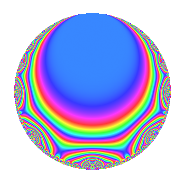# Properties

 Label 804.2.a.fLevel 804 Weight 2 Character orbit 804.a Self dual Yes Analytic conductor 6.420 Analytic rank 0 Dimension 5 CM No Inner twists 1

# Related objects

## Newspace parameters

 Level: $$N$$ = $$804 = 2^{2} \cdot 3 \cdot 67$$ Weight: $$k$$ = $$2$$ Character orbit: $$[\chi]$$ = 804.a (trivial)

## Newform invariants

 Self dual: Yes Analytic conductor: $$6.41997232251$$ Analytic rank: $$0$$ Dimension: $$5$$ Coefficient field: 5.5.24571284.1 Coefficient ring: $$\Z[a_1, \ldots, a_{17}]$$ Coefficient ring index: $$1$$ Fricke sign: $$-1$$ Sato-Tate group: $\mathrm{SU}(2)$

## $q$-expansion

Coefficients of the $$q$$-expansion are expressed in terms of a basis $$1,\beta_1,\beta_2,\beta_3,\beta_4$$ for the coefficient ring described below. We also show the integral $$q$$-expansion of the trace form.

 $$f(q)$$ $$=$$ $$q + q^{3} + ( 1 - \beta_{1} ) q^{5} + ( 1 - \beta_{2} ) q^{7} + q^{9} +O(q^{10})$$ $$q + q^{3} + ( 1 - \beta_{1} ) q^{5} + ( 1 - \beta_{2} ) q^{7} + q^{9} + ( 1 + \beta_{4} ) q^{11} + ( 1 - \beta_{4} ) q^{13} + ( 1 - \beta_{1} ) q^{15} + ( \beta_{2} - \beta_{3} ) q^{17} + ( 2 + 2 \beta_{1} + \beta_{2} + \beta_{3} ) q^{19} + ( 1 - \beta_{2} ) q^{21} + ( \beta_{1} + \beta_{2} + \beta_{3} - \beta_{4} ) q^{23} + ( 2 + \beta_{2} ) q^{25} + q^{27} + ( 1 + \beta_{2} + \beta_{3} - \beta_{4} ) q^{29} + ( -\beta_{2} - 2 \beta_{3} + \beta_{4} ) q^{31} + ( 1 + \beta_{4} ) q^{33} + ( -2 - \beta_{1} - 2 \beta_{2} - 2 \beta_{3} + \beta_{4} ) q^{35} + ( 2 - \beta_{3} + \beta_{4} ) q^{37} + ( 1 - \beta_{4} ) q^{39} + ( 2 + \beta_{1} + 2 \beta_{3} + \beta_{4} ) q^{41} + ( 2 \beta_{1} + \beta_{2} - \beta_{4} ) q^{43} + ( 1 - \beta_{1} ) q^{45} + ( -2 - \beta_{2} + \beta_{3} - 2 \beta_{4} ) q^{47} + ( 5 - \beta_{2} + 2 \beta_{3} + \beta_{4} ) q^{49} + ( \beta_{2} - \beta_{3} ) q^{51} + ( -2 + \beta_{1} - \beta_{4} ) q^{53} + ( 3 + 2 \beta_{3} + \beta_{4} ) q^{55} + ( 2 + 2 \beta_{1} + \beta_{2} + \beta_{3} ) q^{57} + ( -3 + \beta_{1} - \beta_{2} - \beta_{3} ) q^{59} + ( 1 - 2 \beta_{1} - 2 \beta_{2} - 2 \beta_{3} + \beta_{4} ) q^{61} + ( 1 - \beta_{2} ) q^{63} + ( -1 - 2 \beta_{1} - 2 \beta_{3} - \beta_{4} ) q^{65} + q^{67} + ( \beta_{1} + \beta_{2} + \beta_{3} - \beta_{4} ) q^{69} + ( -5 + \beta_{4} ) q^{71} + ( 3 - 2 \beta_{1} - \beta_{3} ) q^{73} + ( 2 + \beta_{2} ) q^{75} + ( -1 - 2 \beta_{1} - 4 \beta_{2} - 2 \beta_{3} + 3 \beta_{4} ) q^{77} + ( -2 \beta_{1} + 2 \beta_{2} ) q^{79} + q^{81} + ( -4 - 3 \beta_{1} - \beta_{4} ) q^{83} + ( 1 - 2 \beta_{1} + 2 \beta_{3} - 3 \beta_{4} ) q^{85} + ( 1 + \beta_{2} + \beta_{3} - \beta_{4} ) q^{87} + ( -2 + 2 \beta_{1} + \beta_{2} - \beta_{3} ) q^{89} + ( 3 + 2 \beta_{1} + 2 \beta_{2} + 2 \beta_{3} - 3 \beta_{4} ) q^{91} + ( -\beta_{2} - 2 \beta_{3} + \beta_{4} ) q^{93} + ( -5 - 2 \beta_{1} + 2 \beta_{2} + 2 \beta_{3} + \beta_{4} ) q^{95} + ( 3 - 2 \beta_{1} + 2 \beta_{2} - 3 \beta_{4} ) q^{97} + ( 1 + \beta_{4} ) q^{99} +O(q^{100})$$ $$\operatorname{Tr}(f)(q)$$ $$=$$ $$5q + 5q^{3} + 3q^{5} + 5q^{7} + 5q^{9} + O(q^{10})$$ $$5q + 5q^{3} + 3q^{5} + 5q^{7} + 5q^{9} + 6q^{11} + 4q^{13} + 3q^{15} + q^{17} + 13q^{19} + 5q^{21} + 10q^{25} + 5q^{27} + 3q^{29} + 3q^{31} + 6q^{33} - 9q^{35} + 12q^{37} + 4q^{39} + 11q^{41} + 3q^{43} + 3q^{45} - 13q^{47} + 24q^{49} + q^{51} - 9q^{53} + 14q^{55} + 13q^{57} - 12q^{59} + 4q^{61} + 5q^{63} - 8q^{65} + 5q^{67} - 24q^{71} + 12q^{73} + 10q^{75} - 4q^{77} - 4q^{79} + 5q^{81} - 27q^{83} - 4q^{85} + 3q^{87} - 5q^{89} + 14q^{91} + 3q^{93} - 30q^{95} + 8q^{97} + 6q^{99} + O(q^{100})$$

Basis of coefficient ring in terms of a root $$\nu$$ of $$x^{5} - 2 x^{4} - 15 x^{3} + 10 x^{2} + 64 x + 38$$:

 $$\beta_{0}$$ $$=$$ $$1$$ $$\beta_{1}$$ $$=$$ $$\nu$$ $$\beta_{2}$$ $$=$$ $$\nu^{2} - 2 \nu - 6$$ $$\beta_{3}$$ $$=$$ $$($$$$\nu^{4} - 5 \nu^{3} - 8 \nu^{2} + 34 \nu + 34$$$$)/4$$ $$\beta_{4}$$ $$=$$ $$($$$$\nu^{4} - 3 \nu^{3} - 10 \nu^{2} + 18 \nu + 28$$$$)/2$$
 $$1$$ $$=$$ $$\beta_0$$ $$\nu$$ $$=$$ $$\beta_{1}$$ $$\nu^{2}$$ $$=$$ $$\beta_{2} + 2 \beta_{1} + 6$$ $$\nu^{3}$$ $$=$$ $$\beta_{4} - 2 \beta_{3} + \beta_{2} + 10 \beta_{1} + 9$$ $$\nu^{4}$$ $$=$$ $$5 \beta_{4} - 6 \beta_{3} + 13 \beta_{2} + 32 \beta_{1} + 59$$

## Embeddings

For each embedding $$\iota_m$$ of the coefficient field, the values $$\iota_m(a_n)$$ are shown below.

For more information on an embedded modular form you can click on its label.

Label $$\iota_m(\nu)$$ $$a_{2}$$ $$a_{3}$$ $$a_{4}$$ $$a_{5}$$ $$a_{6}$$ $$a_{7}$$ $$a_{8}$$ $$a_{9}$$ $$a_{10}$$
1.1
 3.96721 2.95721 −0.789611 −1.65253 −2.48228
0 1.00000 0 −2.96721 0 −0.804360 0 1.00000 0
1.2 0 1.00000 0 −1.95721 0 4.16931 0 1.00000 0
1.3 0 1.00000 0 1.78961 0 4.79729 0 1.00000 0
1.4 0 1.00000 0 2.65253 0 0.964067 0 1.00000 0
1.5 0 1.00000 0 3.48228 0 −4.12631 0 1.00000 0
 $$n$$: e.g. 2-40 or 990-1000 Embeddings: e.g. 1-3 or 1.5 Significant digits: Format: Complex embeddings Normalized embeddings Satake parameters Satake angles

## Inner twists

This newform does not admit any (nontrivial) inner twists.

## Atkin-Lehner signs

$$p$$ Sign
$$2$$ $$-1$$
$$3$$ $$-1$$
$$67$$ $$-1$$

## Hecke kernels

This newform can be constructed as the kernel of the linear operator $$T_{5}^{5} - 3 T_{5}^{4} - 13 T_{5}^{3} + 37 T_{5}^{2} + 36 T_{5} - 96$$ acting on $$S_{2}^{\mathrm{new}}(\Gamma_0(804))$$.